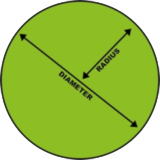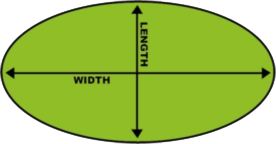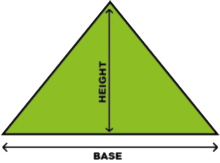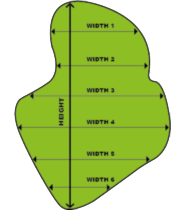## This guide helps you work out the metres of instant lawn you need

Once you have the correct m2 contact us and we will give you a quote. It is advisable to allow extra turf when ordering as you will have some wastage particularly if your lawn is of an irregular shape.

## Measuring a rectangleMeasure the height and width of the square or rectangular area in metres.

To calculate the amount of turf required, multiply height x width

Example:
height 6m; width 15m
6m x 15m= 90m2

## Measuring a circleMeasure the diameter of the circular area in metres.

To calculate the amount of turf required, use this simple formula.      r = radius   d = diameter

r  x  r  x  3.14

Example:
6.5m x 6.5m x 3.14  = 132.7m

## Measuring an ovalMeasure the length and width of the oval area in metres.

To calculate the amount of turf required, multiply length x 0.80 then by width.

Example:
length 19m; width 10m
19m x 0.80 = 15.2m
15.2m x 10m = 152m2

## Measuring a triangleMeasure the base and height of the triangular area in metres.

To calculate the amount of turf required, multiply base x 0.50 then by height.

Example:
base 16m; height 9m
16m x 9m ÷ 2 = 72m2

## Measuring unusual shapesMeasure the metres by firstly dividing area into sections of regular shapes i.e.: circle, square and triangle.

To calculate the required amount of turf in square metres, apply the formulas for each shape and then add together to form total area. This calculation is only a rough guide; it is quite hard to determine the correct measurements for such an unusual area.

Example:
length 13.5m
width 1 = 3m; width 2 = 2.5m; width 3 = 4m; width 4 = 4m; width 5 = 3.5m; width 6 = 1.8m;
3m + 2.5m + 4m + 4m + 3.5m 1.8m = 18.8m
18.8m/6(total of widths measured) = 3.13m
3.13 x 13.5m (length) = 42.25m3

## How to measure your lawn video

Start typing and press Enter to search